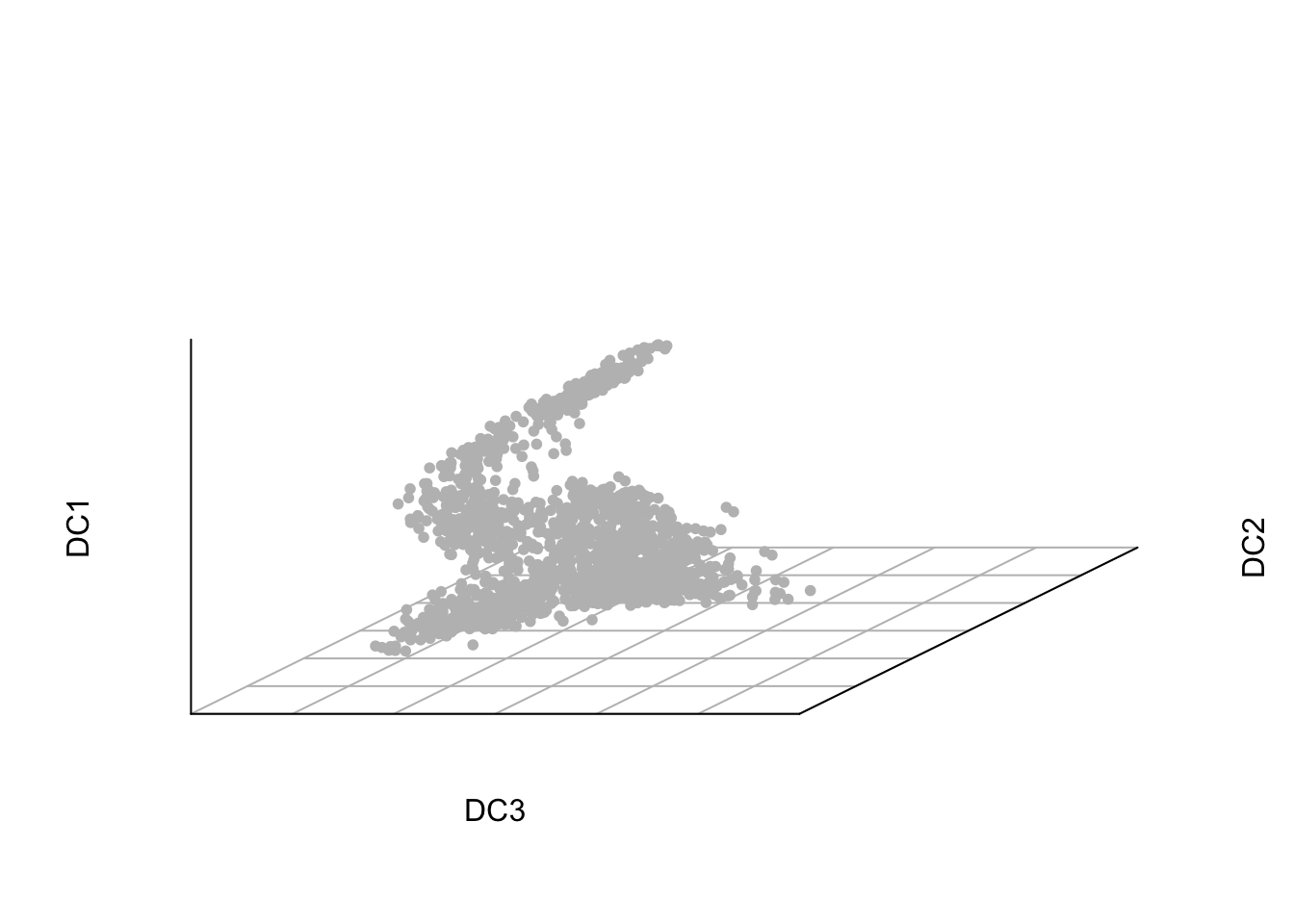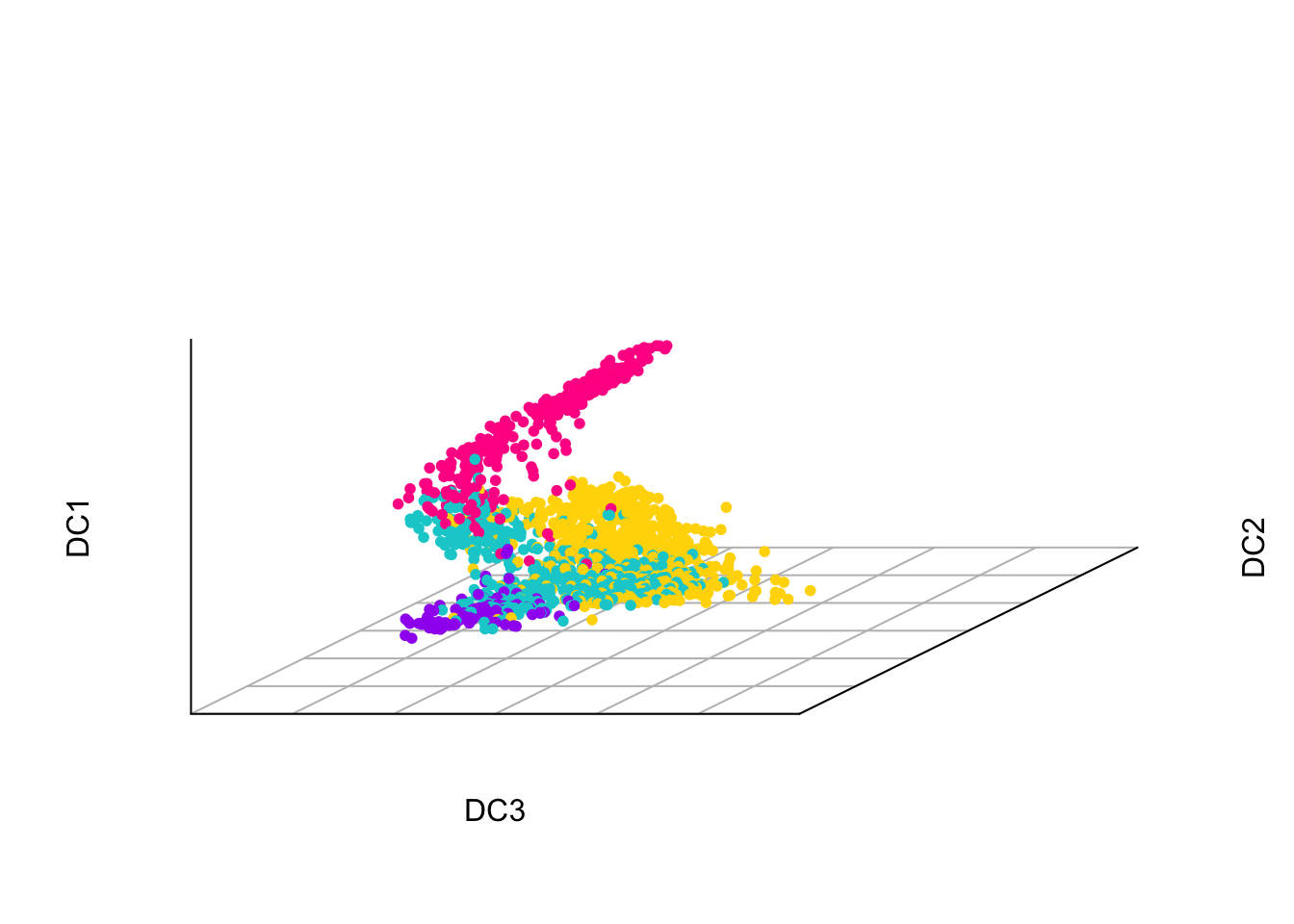This is a demonstration of how to project new data sets onto the diffusion map of adult haematopoiesis. This projection is performed in the R programming environment. You will need the destiny package installed. This can be downloaded from Bioconductor.

``library(destiny)``

The next step is to load the data for the 1,656 blood stem and progenitor cells. These can be downloaded from http://blood.stemcells.cam.ac.uk/single_cell_atlas.html. Rows should be labelled with Ensembl gene IDs and columns with cell names.

``````originalExpressionMatrix <- read.table('normalisedCountsVariableGenes.txt', header = T, row.names = 1)
originalExpressionMatrix[1:5,1:5]``````
``````##                     HSPC_025 HSPC_031 HSPC_037 LT.HSC_001 HSPC_001
## ENSMUSG00000030159  0.000000   0.0000   0.0000     0.0000   0.0000
## ENSMUSG00000053470 28.683808 116.5984   0.0000     0.0000   0.0000
## ENSMUSG00000041729  1.687283   0.0000 119.5414   288.6915 710.9228
## ENSMUSG00000048489  0.000000   0.0000   0.0000     0.0000   0.0000
## ENSMUSG00000046080  0.000000   0.0000   0.0000     0.0000   0.0000``````

We now perform the diffusion map dimensionality reduction on these data using functions from the destiny package. The log transformation acts as a variance stabilising transformation. Here for simplicity we have used the plot function to plot the diffusion maps. To alter the viewing angle or interactively rotate the 3D plot, packages such as rgl and plot3D can be used.

``````logOriginalExpression <- log2(originalExpressionMatrix + 1)
dm <- DiffusionMap(t(logOriginalExpression), distance = "cosine", sigma = .16)
plot(dm, c(3,2,1), pch=20, col="grey")``````The diffusion map can be coloured in by features such as cluster ID.Next we load and prepare the new data set that we wish to project. Here we are demonstrating projection of the data from (Grover et al., 2016). The normalised expression matrix for this demonstration can be downloaded from http://blood.stemcells.cam.ac.uk/single_cell_atlas.html, or you can use your own data for projection.

``newExpressionMatrix <- read.table('grover_expression.txt', header = T)``

To perform the projection we require both new and old data to have the same set of genes. Then we can perform the projection using the dm.predict function from the destiny package.

``````newExpressionMatrix <- newExpressionMatrix[rownames(originalExpressionMatrix), ]
logNewExpression <- log2(newExpressionMatrix + 1)
dmProject <- dm.predict(dm, t(logNewExpression))
plot(dm, c(3,2,1), col = "grey", new.dcs=dmProject, pch=20, col.new = "red")``````